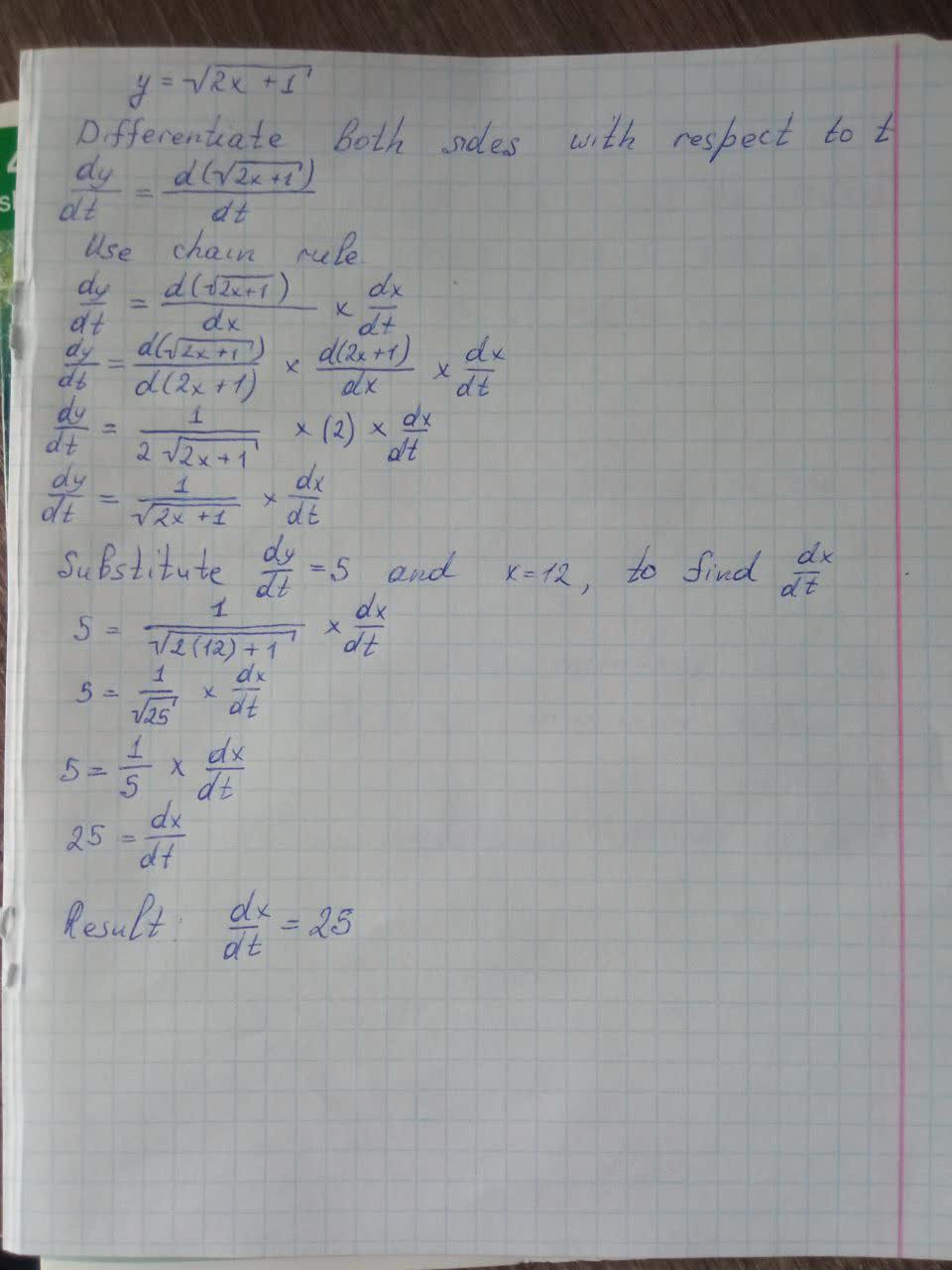Question# Suppose y=\sqrt{2x}+1, where x and y are functions of r.(a) If \frac{dx}{dt}=3, find \frac{dy}{dt} when x = 4.(b) If \frac{dy}{dt}=5, find \frac{dx}{dt} when x = 12.

Derivatives
ANSWEREDSuppose $$y=\sqrt{2x}+1$$, where x and y are functions of r.
(a) If $$\frac{dx}{dt}=3$$, find $$\frac{dy}{dt}$$ when $$x = 4$$.
(b) If $$\frac{dy}{dt}=5$$, find $$\frac{dx}{dt}$$ when $$x = 12$$.